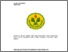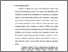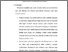# HUBUNGAN ANTARA PEMBERIAN PENGUATAN (REINFORCEMENT) DENGAN MOTIVASI BELAJAR SISWA KELAS XI AKUNTANSI SMK NEGERI 50 JAKARTA

NUGROHO, AGAM (2016) HUBUNGAN ANTARA PEMBERIAN PENGUATAN (REINFORCEMENT) DENGAN MOTIVASI BELAJAR SISWA KELAS XI AKUNTANSI SMK NEGERI 50 JAKARTA. S1 thesis, Universitas Negeri Jakarta.Preview
Text
Cover.pdf

Download (0B) | PreviewPreview
Text
Table_Of_Content.pdf

Download (0B) | PreviewPreview
Text
Chapter 1.pdf

Download (0B) | PreviewText
Chapter 2.pdf
Restricted to Registered users only

Download (0B)Preview
Text
Chapter 3.pdf

Download (0B) | PreviewText
Chapter 4.pdf
Restricted to Registered users only

Download (0B)Preview
Text
Chapter 5.pdf

Download (0B) | PreviewPreview
Text
Bibiliography.pdf

Download (0B) | Preview

## Abstract

AGAM NUGROHO. 8105117987. The Correlation between Reinforcement with Learning Motivation in Students Grade 11 th Accounting of SMK Negeri 50 Jakarta. Study Program of Economic Education, Concentration in Accounting Education, Department of Economic and Administration, Faculty of Economic, State University of Jakarta. 2015. This research aims to determine whether there is the correlation between reinforcement with learning motivation in students grade 11 th Accounting of SMK Negeri 50 Jakarta. This research was conducted for three months since Sptember until January 2016. The method used was survey method with the correlational approach. The populations in this research were all of the students in SMK Negeri 50 Jakarta. The affordable. Populations were all of the 11 th accounting grade’s students as much as 72 students. Proportional random sampling was used to take the samples, there were 61 samples. The data of Reinforcement (Variable X) and Learning Motivation (Variable Y) are primary data that obtained from questionnaires using likert scale model. The regression equation is Ŷ = 51,95 + 0,359 X. Test requirements analysis that estimates the error normality test regression of Y on X with the test Liliefors produce L = 0,089 and L = 0,113. Therefore, the data were normally distributed because L table count (0,089) < L (0,113). Linearity test regression produces F count (0,69) < F table table (1,90), meaning that the linear regression equation. Significance of the regression test produces F count = 17,58 and F = 4,02, because F count (17,58) > F (4,02) then the regression equation significantly. Product moment coefficient from pearson produces r table = 0,479, further test correlation coefficient using the t test and the resulting are t = 3,80 and t table = 1,671. Thus it can be stated that the correlation coefficient r = 0,479 is significant. The coefficient of determination obtained by 29.94% which is indicates that the learning motivation determined variation of reinforcement and the remaining 77,06% influenced by other variables are not examined. Based on the research concluded that there is a positive and significant correlation between reinforcement with learning motivation in Students Grade 11 th Accounting of SMK Negeri 50 Jakarta

Item Type: Thesis (S1) Pembimbing I: Dra. Sri Zulaihati, M.Si Pembimbing II:Erika Takidah, SE, M.Si Ilmu Sosial (Social Science) > Pendidikan (Education)Ilmu Sosial (Social Science) > Pendidikan (Education) > Pendidikan Sekolah Lanjutan (Secondary Education) Fakultas Ekonomi > S1 Pendidikan Akuntansi Ahmad Afandi 16 Dec 2017 03:41 16 Dec 2017 03:41 http://repository.fe.unj.ac.id/id/eprint/1415

### Actions (login required)View Item